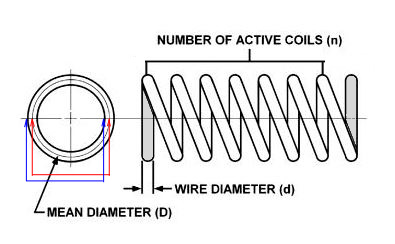Spring Rate Calculator (scroll down)

You can purchase an expensive spring calculator, or you can roll your own, but the easiest way to check your race springs is here...
Use my calculator to determine the base-line Spring Rate for all the springs you have in inventory.

Spring Rate
: The amount of force it takes to compress the spring 1-inch and is expressed in load per inch.

The lower the rate, the softer the spring.  If the front of your racecar is diving on turn entry you can replace the sprng with a heavier load, or increase the shock compression rate.

Spring Load is the amount of weight the spring is designed to carry at a certain height. This is also called the Design Load or Load Rate.
The total un-sprung weight is all the components located below the spring and includes 1/2 the spring's mass in pound weight.

Load Rate should not be confused with Spring Rate

• Our spring is rated at 300 LB/in and was designed for a 3-Inch deflection (compression)

• When deflected (compressed) 3-inches the spring will support 900 lbs. If the load rate is exceeded, the spring is in a bind.

• Therefore, our spring has a Rate of 900 lbs. A Load Rate of 300 lbs per inch times 3 inches. This spring will be tagged as 900 pounds.

Unsprung Weight is the weight of the tires, wheels, knuckles, hubs, axles, and half the weight of the springs, shocks, control arms, and/or links.

Sprung Weight is the weight of the body, chassis, drivetrain, tools, parts and the other half of the total weight of the springs, shocks, control arms and/or links.

Wheel Rate is the spring rate actually measured at the wheel (or tire).  The wheel rate is usually lower than the true spring rate due to factors such as spring position and control arm or axle leverage that can effectively lessen the spring rate at the wheel versus the actual spring rate at the spring.  If you move the spring closer to the tire (and the spring travels parallel to the wheel), the wheel rate and spring rate will become almost the same.

Variable Rate Springs have a soft initial spring rate and to absorb the subtle irregularities of the road/trail progressing to a firmer rate to handle large bumps.
These springs increase in rate as they are compressed. Not very useful on dirt racecars.

Active Coils are the number of coils that do not touch the spring perch (grey area).
FYI: Multiply the spring diameter by the number of coils for the collapsed rate. Subtract this number from the overall length and multiply by two.This is your shock travel allowance.
(d times n = 6.125 - 10 = 3.875 times 1.5) Your shock will travel 5.8125 inches, you should select a shock that travels a minimum of 7 inches.

Increase Effect On Rate Chart:  Required Rate = (Force x Travel)/2
This calculation can be used to determine the required spring rate for one corner.
Suggested rate equals sprung weight, less un-sprung weight, multiplied by spring travel, divided by two.

Example: ((750 - 95 = 655) x 2.6)  = 1441 / 2 = 720.5. Round the rate down to 700 and increase the shock compression rate.
Note: Front springs for GM Metric front clip will average 7 active coils with .75 diameter wire.
Note: Rear springs for GM Metric on 9 inch Ford axle will average 12 active coils with .5 diameter wire.

Calculating Spring Criteria:

Increase wire diameter (d) - increase the spring rate. Increase coil diameter (D) - decrease the spring rate. Increase active coils (n) - decrease the spring rate.

 Coil Spring Rate Calculator:
 All Measurements in inches - Enter as decimal - Zero all Fields before entry For Inch Fractions To Decimal Chart, Click HEREExample: 0.875 Wire Diameter - 5 inch Mean Diameter - Number of Coils = 7 (click calculate)

 Coil Spring Wire Diameter (d) Coil Spring Mean Diameter (D) Number of Active Coils (n) = Coil Spring Rate (lb per in)

Use this calculator to determine your springs based upon dimensions only. Remember; tag your springs.
The spring material is not considered here because most racing springs are carbon steel wire.

Thank you and enjoy.